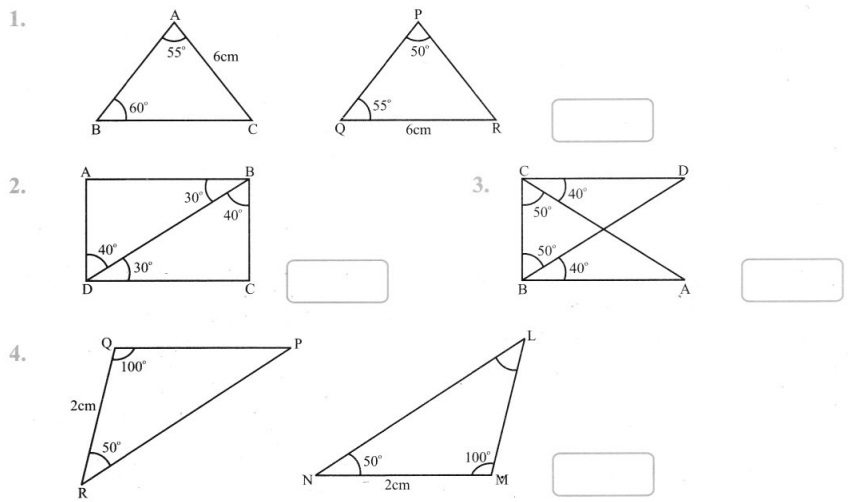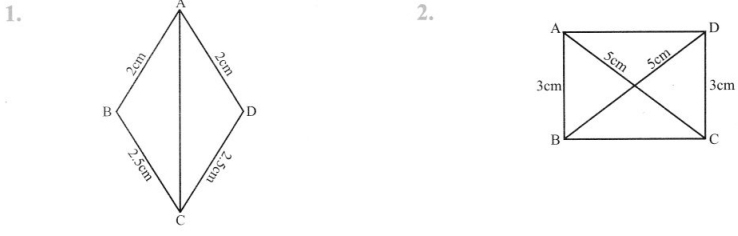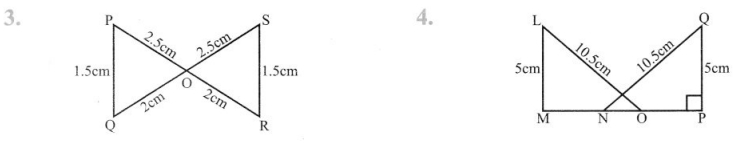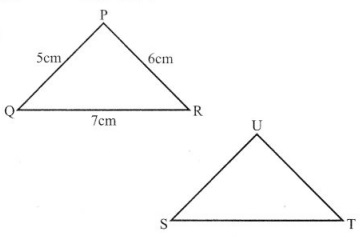# Grade 7 Congruence of Triangles Worksheets

### Class 7 Maths Congruence of Triangles Multiple Choice Questions (MCQs)

1. Two angles are congruent if they have:
(a) Same name
(b) Equal measures
(c) Unequal measures
(d) None of these.

2. Which of the following is not a congmence criterion?
(a) SSS
(b) SAS
(c) ASA
(d) None of these

3. If a ∆ ABC ≅ ∆ PQR, then AB is equal to:
(a) QR
(b) PQ
(c) PR
(d) None of these

4. In ∆ ABC and ∆PQR, AB = 4cm, BC = 5cm, AC = 6cm and PQ = 4cm, QR = 5cm, PR = 6cm, then which of the following is true:
(a) ∆ ABC ≅ ∆ QRP
(b) ∆ ABC ≅ ∆ PQR
(c) ∆ ABC ≅ ∆ PRQ
(d) ∆ ABC ≅ ∆ QPR

5. If ∆ DEF ≅ ∆ BCA, then the part of ∆ BCA that correspond to ∠E is:
(a) ∠A
(b) ∠B
(c) ∠C
(d) none of these

6. If ∆ DEF ≅ ∆ ACB, then the part of ∆ ACB that correspond to ∠F is:
(a) ∠A
(b) ∠B
(c) ∠C
(d) none of these

7. ∆ ABC and ∆ PQR are congruent under the correspondence: ABC ↔ RQP, then the part of AABC that correspond to ∠P is:
(a) ∠A
(b) ∠B
(c) ∠C
(d) none of these

8. ∆ ABC and ∆ PQR are congruent under the correspondence: ABC ↔ RQP, then the part of ∆ ABC that correspond to ∠Q is:
(a) ∠A
(b) ∠B
(c) ∠C
(d) none of these

9. ∆ ABC and ∆ PQR are congruent under the correspondence: ABC ↔ RPQ, then the part of ∆ ABC that correspond to$\overline{P Q}$ is:
(a)$\overline{A B}$
(b)$\overline{B C}$
(c)$\overline{C A}$
(d) none of these

10. ∆ ABC and ∆ PQR are congruent under the correspondence: BCA ↔ RPQ, then the part of ∆ ABC that correspond to$\overline{P Q}$ is:
(a)$\overline{A B}$
(b)$\overline{B C}$
(c)$\overline{C A}$
(d) none of these

11. ∆ ABC and ∆ PQR are congruent under the correspondence: BCA ↔ RPQ, then the part of ∆ ABC that correspond to$\overline{P R}$ is:
(a)$\overline{A B}$
(b)$\overline{B C}$
(c)$\overline{C A}$
(d) none of these

12. What is the side included between the angles A and B in ∆ ABC?
(a)$\overline{A B}$
(b)$\overline{B C}$
(c)$\overline{C A}$
(d) none of these

13. ∆ ABC is right triangle in which ∠A = 90° and AB = AC. The values of ∠B and ∠C will be:
(a) ∠B = ∠C = 60°
(b) ∠B = ∠C = 30°
(c) ∠B = ∠C = 45°
(d) ∠B = ∠C = 50°

14. If the vertical angle of a isosceles triangle is 40° then measure of other two angles will be:
(a) 60° 60°
(b) 70° 70°
(c) 50 50°
(d) 75° 75°

15. Which one of the following is the value of congruency?
(a) SAS
(b) ASS
(c) SSA
(d) none of these

16. What is the angle included between the sides PN and PM of AMNP?
(a) ∠N
(b) ∠P
(c) ∠M
(d) none of these

17. In triangles ABC and PQR, AB = 3.5 cm, BC = 7.1 cm, AC = 5 cm, PQ = 7.1 cm, QR = 5 cm and PR = 3.5 cm, then which of the following is true?
(a) ∆ ABC ≅ ∆ QRP
(b) ∆ ABC ≅ ∆ PQR
(c) ∆ ABC ≅ ∆ RPQ
(d) ∆ ABC ≅ ∆ QPR

18. In triangles ABC and DEF. AB = 7 cm. BC = 5 cm, ∠B = 50°, DE = 5 cm, EF = 7 cm, ∠E = 50° By which congruence rule the triangles are congruent?
(a) SAS
(b) RHS
(c) ASA
(d) SSS

19. It is to be established by RHS congruence rule that ∆ ABC ≅ ∆ RPQ. What additional information is needed, if it is given that ∠B = ∠P = 90° and AB = RP?
(a) AC = RQ
(b) ∠A = ∠P
(c) BC = QR
(d) none of these

20. If ABC and DEF are congruent triangles such that ∠A = 47° and ∠E = 83°, then ∠C =
(a) 50°
(b) 60°
(c) 70°
(d) 80°

Class 7 Maths Congruence of Triangles If ∆ PQR and ∆ LMN are congruent under the correspondence QPR ↔ LMN then fill up the blanks:

1. ∠R = ………………
2. ∠P = ………………
3. ∠Q = ……………..
4. ∠QR = …………..
5. ∠QP = ……………
6. ∠RP = ………………

### Class 7 Maths Congruence of Triangles True(T) And False(F)

1. If three corresponding angles of two triangles are equal then triangles are congruent.
2. The congruent figure super impose each other completely.
3. The top and bottom faces of a kaleidoscope are congruent.
4. Two figure are congruent, if both have the same shape.

### Class 7 Maths Congruence of Triangles Very Short Answer Type Questions

In each of the given triangles applying only ASA congruence criterion, determine which triangles are congruent. Also write the congruent triangles in symbolic form.### Class 7 Maths Congruence of Triangles Short Answer Type Questions

In each of the given pair of triangles applying only SSS congruence criterion, determine which pairs of triangles are congruent. Also write the congruent triangles in symbolic form:### Class 7 Maths Congruence of Triangles Long Answer Type Questions

If ∆ PQR is congruent to ∆ STU then what is the length of TU?

1.2. A tree is broken at a height of 5m from the ground and its top touches the ground at a distance of 12m from the base of the tree. Find the original height of the tree.
3. The diagonals of a rhombus measure 16cm and 30cm. Find its perimeter.